### 13.4.1.1 Calculus of variations

Lagrangian mechanics is based on the calculus of variations, which is the subject of optimization over a space of paths. One of the most famous variational problems involves constraining a particle to travel along a curve (imagine that the particle slides along a frictionless track). The problem is to find the curve for which the ball travels from one point to the other, starting at rest, and being accelerated only by gravity. The solution is a cycloid function called the Brachistochrone curve . Before this problem is described further, recall the classical optimization problem from calculus in which the task is to find extremal values (minima and maxima) of a function. Let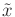denote a smooth function fromto, and let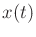denote its value for any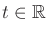. From standard calculus, the extremal values ofare allfor which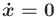. Suppose that at some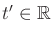,achieves a local minimum. To serve as a local minimum, tiny perturbations of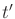should result in larger function values. Thus, there exists some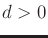such that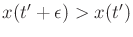for any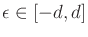. Each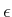represents a possible perturbation of.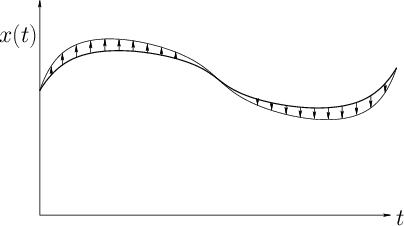The calculus of variations addresses a harder problem in which optimization occurs over a space of functions. For each function, a value is assigned by a criterion called a functional.13.10 A procedure analogous to taking the derivative of the function and setting it to zero will be performed. This will be arrived at by considering tiny perturbations of an entire function, as opposed to theperturbations mentioned above. Each perturbation is itself a function, which is called a variation. For a function to minimize a functional, any small enough perturbation of it must yield a larger functional value. In the case of optimizing a function of one variable, there are only two directions for the perturbation: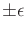. See Figure 13.12. In the calculus of variations, there are many different directions'' because of the uncountably infinite number of ways to construct a small variation function that perturbs the original function (the set of all variations is an infinite-dimensional function space; recall Example 8.5).

Letdenote a smooth function from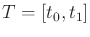into. The functional is defined by integrating a function over the domain of. Let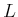be a smooth, real-valued function of three variables,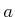,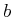, and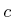.13.11 The arguments ofmay be any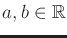and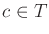to yield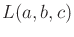, but each has a special interpretation. For some smooth function,is used to evaluate it at a particular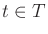to obtain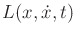. A functional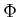is constructed usingto evaluate the whole functionas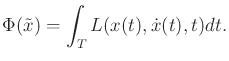(13.114)

The problem is to select anthat optimizes. The approach is to take the derivative ofand set it equal to zero, just as in standard calculus; however, differentiatingwith respect tois not standard calculus. This usually requires special conditions on the class of possible functions (e.g., smoothness) and on the vector space of variations, which are implicitly assumed to hold for the problems considered in this section.

Example 13..9 (Shortest-Path Functional)   As an example of a functional, consider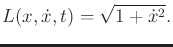(13.115)

When evaluated on a function, this yields the arc length of the path.Another example of a functional has already been seen in the context of motion planning. The cost functional (8.39) assigns a cost to a path taken through the state space. This provided a natural way to formulate optimal path planning. A discrete, approximate version was given by (7.26).

Let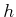be a smooth function over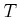, and let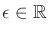be a small constant. Consider the function defined as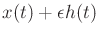for all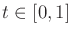. If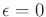, then (13.114) remains the same. Asis increased or decreased, then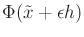may change. The functionis like the direction'' in a directional derivative. If for any smooth function, their exists some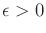such that the valueincreases, thenis called an extremal of. Any small perturbation tocauses the value ofto increase. Therefore,behaves like a local minimum in a standard optimization problem.

Let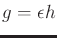for someand function. The differential of a functional can be approximated as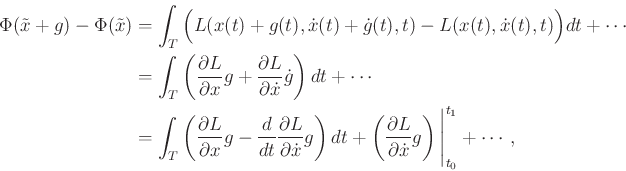(13.116)

in which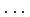represents higher order terms that will vanish in the limit. The last step follows from integration by parts: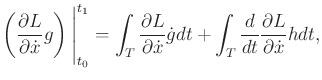(13.117)

which is just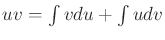. Consider the value of (13.116) asbecomes small, and assume that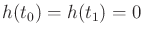. Forto be an extremal function, the change expressed in (13.116) should tend to zero as the variations approach zero. Based on further technical assumptions, including the Fundamental Lemma of the Calculus of Variations (see Section 12 of ), the Euler-Lagrange equation,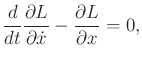(13.118)

is obtained as a necessary condition forto be an extremum. Intuition can be gained by studying the last line of (13.116). The integral attains a zero value precisely when (13.118) is satisfied. The other terms vanish because, and higher order terms disappear in the limit process.

The partial derivatives ofwith respect to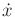andare defined using standard calculus. The derivative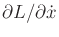is evaluated by treatingas an ordinary variable (i.e., as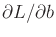when the variables are named as in). Following this, the derivative ofwith respect to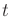is taken. To illustrate this process, consider the following example.

Example 13..10 (A Simple Variational Problem)   Letbe a functional defined as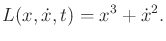(13.119)

The partial derivatives with respect toandare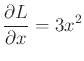(13.120)

and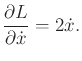(13.121)

Taking the time derivative of (13.121) yields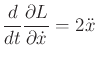(13.122)

Substituting these into the Euler-Lagrange equation (13.118) yields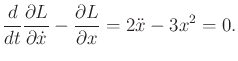(13.123)

This represents a second-order differential constraint that constrains the acceleration as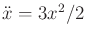. By constructing a 2D phase space, the constraint could be expressed using first-order differential equations.Steven M LaValle 2020-08-14